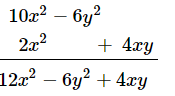# The two adjacent sides of a rectangle are

Question:

The two adjacent sides of a rectangle are 5x2 − 3y2 and x2 + 2xy. Find the perimeter.

Solution:

Sides of the rectangle are $l$ and $b$.

$l=5 x^{2}-3 y^{2}$

$b=x^{2}+2 x y$

Perimeter of the rectangle is $(2 l+2 b)$.

Perimeter $=2\left(5 x^{2}-3 y^{2}\right)+2\left(x^{2}+2 x y\right)$

$=10 x^{2}-6 y^{2}+2 x^{2}+4 x y$Hence, the perimeter of the rectangle is $12 x^{2}-6 y^{2}+4 x y$.